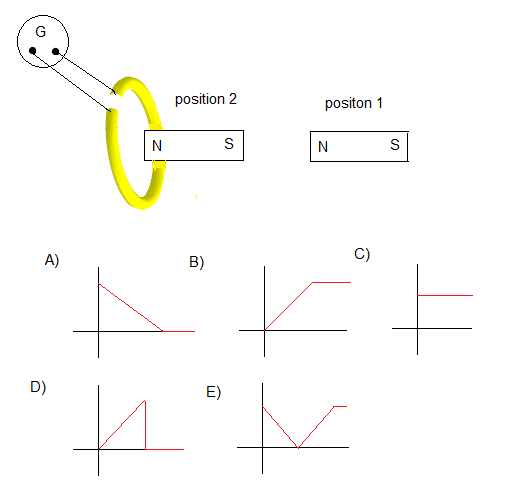# SAT Physics Practice Questions with Solutions Electromagnetism

Sat Physics subject questions on electromagnetism similar to the questions in the SAT test are presented with detailed solutions and explanations.

### Question 1

The primary of a transformer is connected to a source of voltage that has two components: an alternating current (AC) component of 120 volts and a steady direct current (DC) component of 5 volts. The number of turns of the primary is 300 and the that of the secondary is 6000. What is the voltage at the output of the secondary?
A) 2400 volts AC and 100 volts DC
B) 2400 volts AC only
C) 100 volts DC only
D) 2500 volts AC only
E) 2500 volts DC only

### Question 2

An electric motor transforms
A) mechanical energy into electrical energy
B) electrical energy into mechanical energy
C) Potential energy into electrical energy
D) A high voltage into a smaller voltage
E) A small voltage into a higher voltage

### Question 3

A transformer with an efficiency of 80% is connected to 50-volt alternating current source. The voltage and current in the secondary are 500 Volts and 0.1 Amperes respectively. What is the current in the primary?
A) 0.8 A
B) 1.0 A
C) 1.25 A
D) 1.35 A
E) 1.15 A

### Question 4

A bar of length L slides along electrical wires in a direction perpendicular to a magnetic field B directed out of the page. The bar and part of the wires including the resistor R form a closed circuit. Which of the following will increase the electric current flowing in R?(assume that the resistance due to the wires and the bar is negligible)
(I) decreasing the magnitude of B
(II) moving the bar faster
(III) increasing the length L of the barA) (I)
B) (II) only
C) (III) only
D) (II) and/or (III)
E) (I), (II) and (III)

### Question 5

A permanent magnet is inserted at constant speed inside a loop from left and out of the loop again from the right as shown below. While the magnet is moving from left to right,A) the current in the loop will be from A to B
B) the current in the loop will be from A to B and then from B to A
C) the current in the loop will be from B to A
D) the current in the loop will be from B to A and then from A to B
E) there is no current in the loop

### Question 6

A magnet initially stationary is pushed closer to a loop then stopped. Which of the graphs of the current shown below closely represents the variation of the current in the galvanometer detecting the current in the loop?### Question 7

In the figure below the uniform magnetic field B is parallel to the rectangle. What is the magnetic flux of B through the rectangle?A) 0.025 Wb
B) 0
C) 0.004 Wb
D) 250 Wb
E) 0.01 Wb

### Question 8

Let Vp, Ip and Np be the voltage, the current and the number of turns of the primary of an electric transformer and Vs, Is and Ns be the voltage, the current and the number of turns of its secondary. If this transformer is not 100% efficient, which of the following must be true?
A) Vp = Vs
B) Vs Is > Vp Ip
C) Vs Is = Vp Ip
D) Ip = Is
E) Vs Is < Vp Ip

### Question 9

Which of the following laws may be used to find the direction of the current induced in a loop close to a moving magnet?
A) Kirchhoff's law
C) Joule's law
D) Lenz's law
E) Ohm's law

### Question 10

Which of the following is true about Lenz's law
(i) It obeys Newton's third Law
(II) It obeys the conservation of energy
(III) It may be used to find direction of induced current
A) (I), (II) and (III)
B) (I) and (II) only
C) (I) and (III) ony
D) (I) only
E) (II) ony